#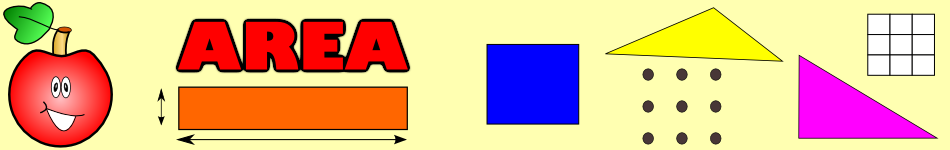There are 366 different Starters of The Day, many to choose from. You will find below some starters on the topic of Area. A lesson starter does not have to be on the same topic as the main part of the lesson or the topic of the previous lesson. It is often very useful to revise or explore other concepts by using a starter based on a totally different area of Mathematics.

Main Page

### Area Starters: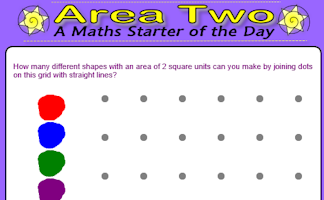How many different shapes with an area of 2 square units can you make by joining dots on this grid with straight lines?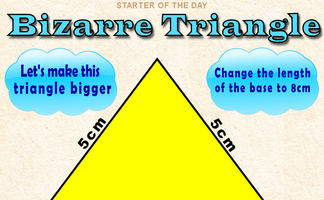By how much would the area of this triangle increase if its base was enlarged to 8cm?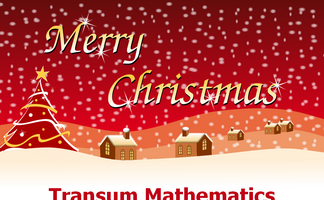Which of the two shapes has the largest area? You will be surprised!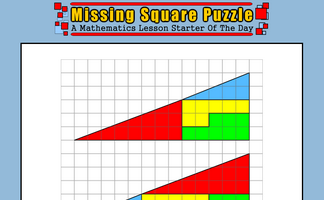The missing square puzzle is an optical illusion used to help students reason about geometrical figures.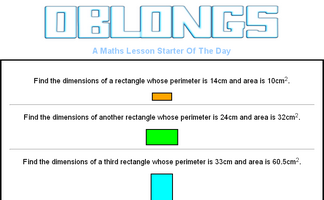Find the dimensions of a rectangle given the perimeter and area.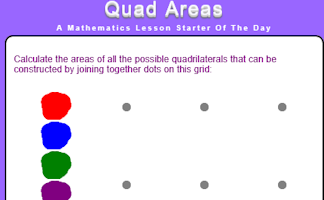Calculate the areas of all the possible quadrilaterals that can be constructed by joining together dots on this grid.

## Exercises#### Area and Perimeter

Show that you know the area and perimeter formulas of basic shapes.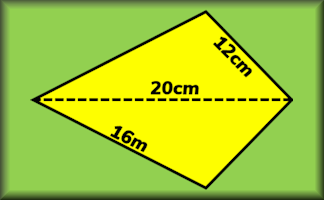#### Area and Perimeter of a Kite

A short exercise to practise using the formulae for area and perimeter of a kite.#### Area and Perimeter of a Parallelogram

Many different ways to practise your skills finding the areas and perimeters of parallelograms.#### Area and Perimeter of a Rectangle

Questions on the areas and perimeters of rectangles which will test your problem solving abilities.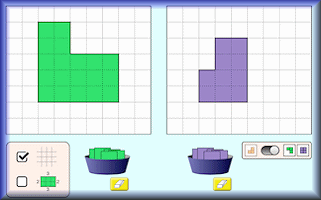#### Area Builder

An interactive workspace in which to make shapes using square tiles with given areas and perimeters.#### Area Maze

Use your knowledge of rectangle areas to calculate the missing measurement of these composite diagrams.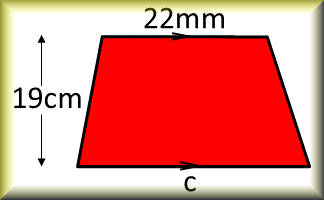#### Area of a Trapezium

Check that you can find the area of a trapezium and use the trapezium area formula for problem solving.#### Area of a Triangle

Calculate the areas of the given triangles in this self marking quiz.#### Area Two

How many different shapes with an area of 2 square units can you make by joining dots on this grid with straight lines?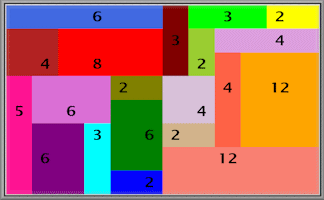#### Area Wall Puzzles

Divide the grid into rectangular pieces so that the area of each piece is the same as the number it contains.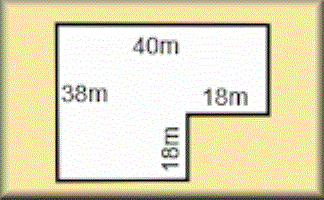#### Areas of Composite Shapes

Find the areas of combined (composite) shapes made up of one or more simple polygons and circles.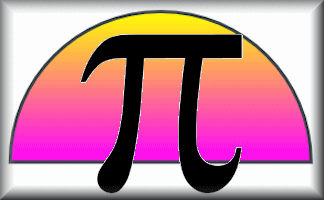#### Circles

Practise using pi to calculate various circle measurements. There are six levels of difficulty.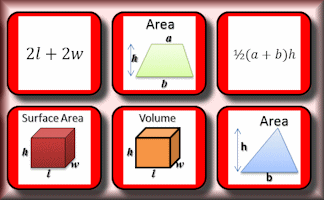#### Formulae Pairs

Find the matching pairs of diagrams and formulae for basic geometrical shapes.#### Pin Board

Rows and columns of dots that can be joined using straight lines to create shapes.Calculate the areas of all the possible quadrilaterals that can be constructed by joining together dots on this grid.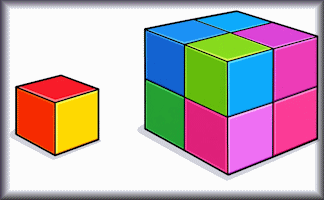#### Similar Shapes

Questions about the scale factors of lengths, areas and volumes of similar shapes.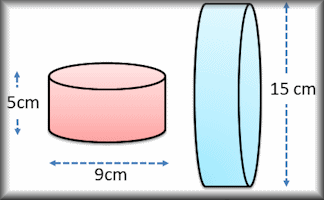#### Surface Area

Work out the surface areas of common solid shapes in this collection of exercises.

### Search

The activity you are looking for may have been classified in a different way from the way you were expecting. You can search the whole of Transum Maths by using the box below.

Have today's Starter of the Day as your default homepage. Copy the URL below then select
Tools > Internet Options (Internet Explorer) then paste the URL into the homepage field.

Set as your homepage (if you are using Internet Explorer)

Do you have any comments? It is always useful to receive feedback and helps make this free resource even more useful for those learning Mathematics anywhere in the world. Click here to enter your comments.For All: